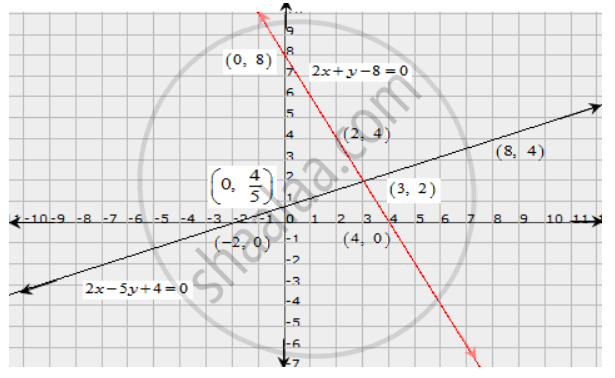Share

# Solve Graphically Each of the Following Systems of Linear Equations. Also, Find the Coordinates of the Points Where the Lines Meet Axis Of Y. 2x − 5y + 4 = 0, 2x + Y − 8 = 0 - CBSE Class 10 - Mathematics

ConceptGraphical Method of Solution of a Pair of Linear Equations

#### Question

Solve graphically each of the following systems of linear equations. Also, find the coordinates of the points where the lines meet axis of y.

2x − 5y + 4 = 0,

2x + y − 8 = 0

#### Solution

The given equations are

2x − 5y + 4 = 0 .............(i)

2x + y − 8 = 0 ..........(ii)

The two points satisfying (i) can be listed in a table as,

 x -2 8 y 0 4

The two points satisfying (ii) can be listed in a table as,

 x 4 2 y 0 4

Now, the graph of equations (i) and (ii) can be drawn as,It is seen that the solution of the given system of equations is given by x = 3, y = 2.

Also, it is observed that the lines (i) and (ii) meet the y-axis at the points (0,4/5) and (0,8) respectively

Is there an error in this question or solution?

#### APPEARS IN

Solution Solve Graphically Each of the Following Systems of Linear Equations. Also, Find the Coordinates of the Points Where the Lines Meet Axis Of Y. 2x − 5y + 4 = 0, 2x + Y − 8 = 0 Concept: Graphical Method of Solution of a Pair of Linear Equations.
S